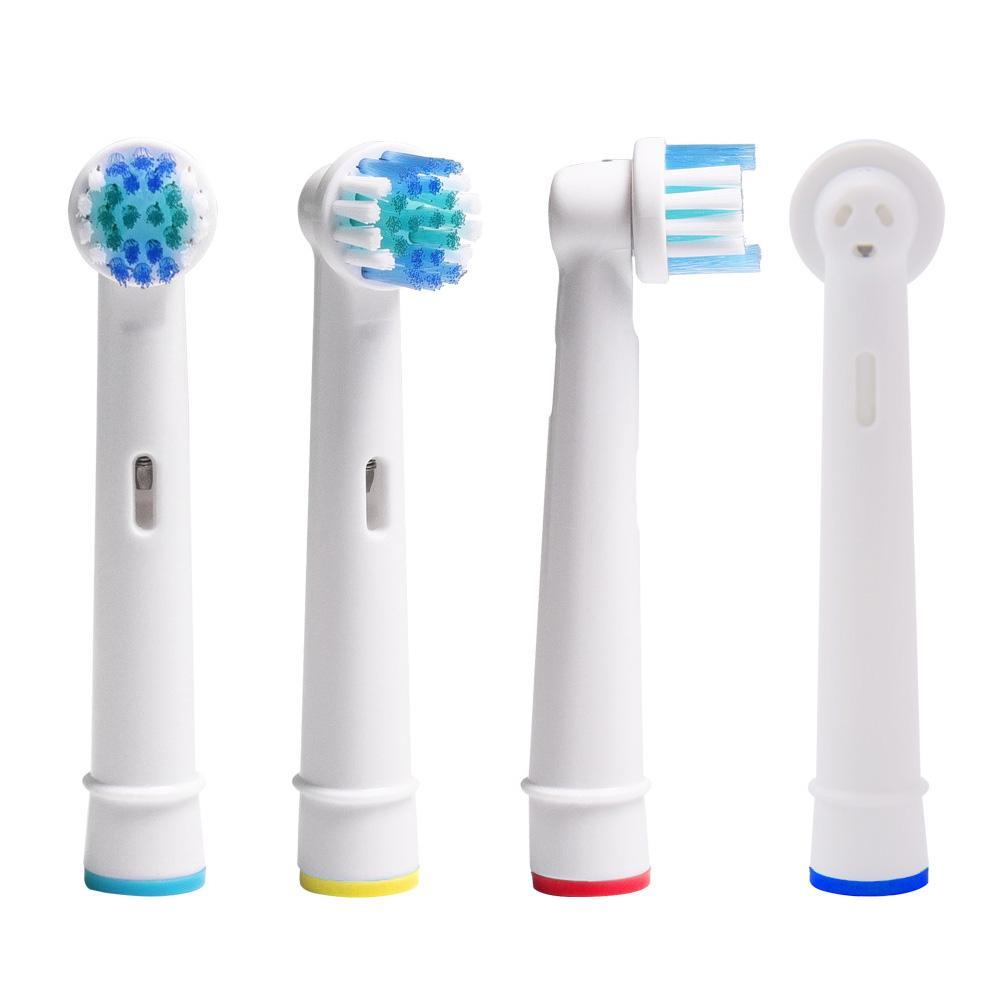#T Ãª t e s d e b r o s s e Ã  d e n t s d e r e c h a n g e 4 p c s p o u r O r a l B A d v a n c e P o w e r H e a l t h 3 D E

Updated Wednesday 17-Jul-2019## T Ãª t e s d e b r o s s e Ã  d e n t s d e r e c h a n g e 4 p c s p o u r O r a l B A d v a n c e P o w e r H e a l t h 3 D E 15

THB 34.28

THB 37.47 Wed 17-Jul-2019

More details at ezbuy.co.th